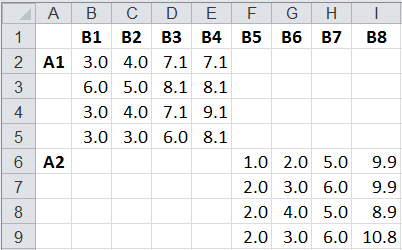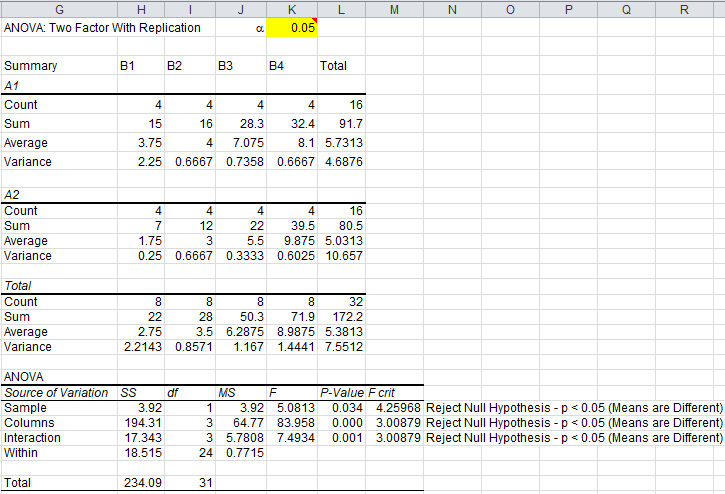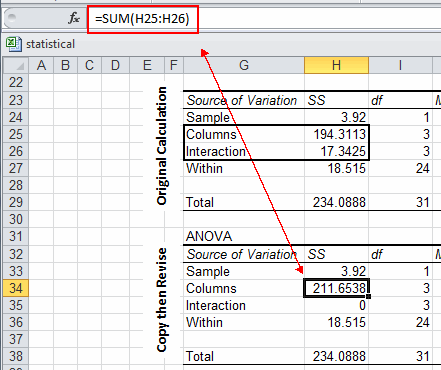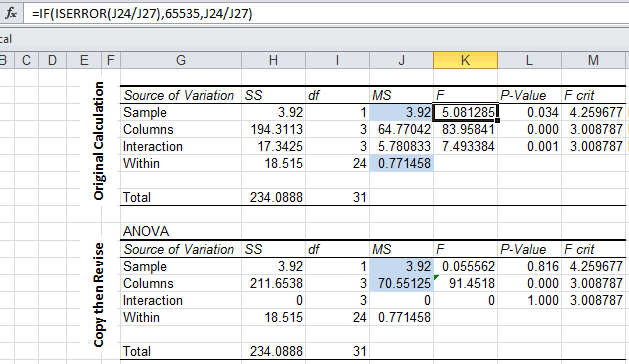# Need to Run Two-Way Nested ANOVA With Replication but Don't Know How?

## QI Macros can run ANOVA tests and interpret the results for you.

### When to Use Two-Way ANOVA for Nested Data

When there are many factors, it can be difficult to "cross" every factor with every other one, so it's possible to use a nested design with Two-Way ANOVA.

### Nested Two-Way ANOVA Analysis With Replication Example

You might have two factors with four replications, but only able to cover each combination (A1 with B1-B4, and A2 with B5-B8) without interaction. So we will need to stack the data and then adjust the results. This example comes from Conrad Carlberg.

Example of Nested ANOVA DataExample of Reorganized Data for Analysis#### First: Perform Two Way ANOVA With Replication using QI Macros

1. Click and drag over cells A1 to E9 of the reorganized data to select it.
2. Click on QI Macros Menu, Statistical Tools and then ANOVA Two Factor with Replication:
3.4. QI Macros will prompt you for how many rows are in each sample (four) and for a significance level. Default is alpha=0.05 for a 95% confidence. QI Macros will perform all of the calculations and interpret the results for you.#### Next, Adjust the Calculations to Account for the Nesting

1. Copy the ANOVA calculations and paste them below the existing calculations:
2. Since there can be no interactions, add the SS values for Columns and Interaction together to eliminate the interaction:3. Then, the F value for A has to be adjusted. See blue shaded cells to determine which values are used to calculate F.4. The adjusted p value for A is now 0.816, not 0.034.